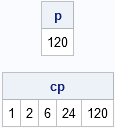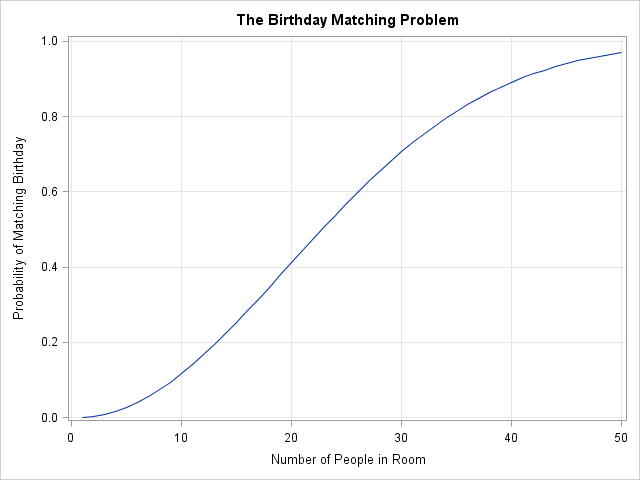The birthday matching problem is a classic problem in probability theory. The part of it that people tend to remember is that in a room of 23 people, there is greater than 50% chance that two people in the room share a birthday. But the birthday matching problem is also a classic problem in computational statistics. I like to use it to emphasize how matrix-vector languages such as SAS/IML, R, and MATLAB can use "vectorized computations" to compute quantities compactly and efficiently. When programmed correctly, you can compute the probabilities in the birthday matching problem without using a single loop.

### Products and cumulative probabilities

A vectorized computation is one in which a function operates on an entire vector of data, rather than on a single scalar quantity. This is efficient because it "pushes" the computation down in to the underlying C or FORTRAN code, rather than requiring the language interpreter to loop over many scalar elements.

For example, the SAS/IML language has functions for computing the product and cumulative product of a vector of values. The PROD function and CUPROD function perform these tasks:

```proc iml; x = 1:5; /* {1 2 3 4 5} */ p = prod(x); /* 5! = 120 */ cp = cuprod(x); print p, cp;```Notice that the CUPROD function, which was introduced in SAS/IML 9.22, computes five answers: the first number, the product of the first two numbers, the product of the first three numbers, and so on. I could compute the same values by calling the PROD function inside a DO loop, but I do not need a loop if I use the CUPROD function. The CUPROD function vectorizes the computation.

The CUPROD function also enables me to compute multiple solutions to the birthday matching problem—without writing a single loop.

### The Birthday Matching Problem

The statement of the birthday matching problem is "If there are N people in a room, what is the chance that two of them have the same birthday?" Every statistical programmer implements the birthday matching problem at some point. Like estimating the value of pi and computing prime numbers, it is a classic problem that is popular because it is easy, fun, and surprising the first time you see the answer. I have used the idea of the birthday matching in several blogs, such as computing the probability that two people at a meeting have the same initials.

I also examine the birthday-matching problem in Chapter 13 of my book, Statistical Programming with SAS/IML Software, both under the assumption that birthdays are uniformly distributed throughout the year and also under an empirical distribution of birthdays by using data from the National Center for Health Statistics (NCHS). The empirical distribution incorporates seasonal effects, as well as the effect of holidays on US births.

### Computing probabilities by using cumulative products

To compute the probabilities in the birthday matching problem, number the people in the room 1, 2, ..., N. Under the assumption that birthdays are uniformly distributed (and that no one in the room was born on leap day, February 29), the probability that the second person does not share a birthday with the first person is 364/365. The probability that the third person’s birthday does not coincide with either of the first two people’s birthdays is 363/365. In general, the probability that the ith person’s birthday does not coincide with any of the birthdays of the first (i-1) people is (365-i+1)/365 = 1-(i-1)/365, for i=1,2,...,365.

Let QN be the probability that no one in the room has a matching birthday. Assuming that that the people in the room are chosen randomly from the population, the birthdays are independent of each other, and QN is just the product of the probabilities for each person. Therefore, the following statements compute the probability of "no matches" in rooms of size N for 1 ≤ N ≤ 50:

```M = 50; /* maximum number of people in room */ i = 1:M; /* enumerate individuals */ iQ = 1 - (i-1)/365; /* individual probabilities */ Q = cuprod(iQ); /* compute probability of "no match" for each room */```

That's it. I've computed 50 probabilities, but there are no loops. In vectorized form, the computation is surprisingly compact. The vectorization works because the probability of no matches in a room with N people is equal to the probability of no matches in a room of (N-1) people, times the factor (1 -(i-1)/365). Therefore the CUPROD function computes the probabilities for 50 rooms in a single function call.

Most of the time people are interested in the probability of a match, which is 1-Q. The following statements use PROC SGPLOT to create the classic graph of the probability of a pair of matching birthdays in a room of N people, N=1,2,...50.

```Prob = 1 - Q; create Birthday var {i Prob}; append; close; quit; /* quit PROC IML */   proc sgplot data=Birthday; series x=i y=Prob; xaxis grid label="Number of People in Room"; yaxis grid label="Probability of Matching Birthday"; title "The Birthday Matching Problem"; run;```As every statistician loves to point out, in a room that contains 23 or more people the chance of a matching birthday exceeds 50%. In a room of 40 or more, the chance of a match is about 90%.

The birthday problem is cool. But along the way I've discussed a general programming principal, vectorization, which is even cooler. The birthday problem is a piece of trivia to keep in the back of your mind, but vectorization is a powerful technique that you can use every day to make your SAS/IML programs more efficient.

Share

### About AuthorDistinguished Researcher in Computational Statistics

Rick Wicklin, PhD, is a distinguished researcher in computational statistics at SAS and is a principal developer of PROC IML and SAS/IML Studio. His areas of expertise include computational statistics, simulation, statistical graphics, and modern methods in statistical data analysis. Rick is author of the books Statistical Programming with SAS/IML Software and Simulating Data with SAS.

### 3 Comments

1.Rather than calling PROD in a DO loop, one could use CUSUM:

proc iml;
x = 1:5; /* {1 2 3 4 5} */
p = exp(cusum(log(x))); /* 5! = 120 */
print p;
quit;